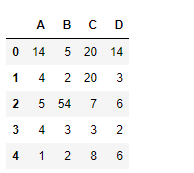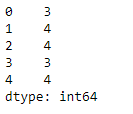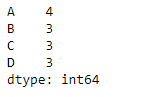Open In App

# Pandas dataframe.nunique() Method

Python is a great language for doing data analysis, primarily because of the fantastic ecosystem of data-centric Python packages. Pandas is one of those packages and makes importing and analyzing data much easier.

## Pandas DataFrame.nunique Syntax

Pandas dataframe.nunique() function returns a Series with a number of distinct observations over the requested axis. If we set the value of the axis to 0, then it finds the total number of unique observations over the index axis. If we set the value of the axis to 1, then it finds the total number of unique observations over the column axis. It also provides the feature to exclude the NaN values from the count of unique numbers.

Syntax: DataFrame.nunique(axis=0, dropna=True)

Parameters:

• axis : {0 or ‘index’, 1 or ‘columns’}, default 0
• dropna : Don’t include NaN in the counts.

Returns : nunique : Series

## Pandas DataFrame nunique() Method

Example 1: Use nunique() function to find the number of unique values over the column axis.

## Python3

 `# importing pandas as pd``import` `pandas as pd` `# Creating the first dataframe``df ``=` `pd.DataFrame({``"A"``:[``14``, ``4``, ``5``, ``4``, ``1``],``                ``"B"``:[``5``, ``2``, ``54``, ``3``, ``2``],``                ``"C"``:[``20``, ``20``, ``7``, ``3``, ``8``],``                    ``"D"``:[``14``, ``3``, ``6``, ``2``, ``6``]})` `# Print the dataframe``df`

Output:Let’s use the dataframe.nunique() function to find the unique values across the column axis.

## Python3

 `# find unique values``df.nunique(axis``=``1``)`

Output:

As we can see in the output, the function prints the total no. of unique values in each row.Example 2: Use nunique() function to find the number of unique values over the index axis in a Dataframe. The Dataframe contains NaN values.

## Python3

 `# importing pandas as pd``import` `pandas as pd` `# Creating the first dataframe``df ``=` `pd.DataFrame({``"A"``: [``"Sandy"``, ``"alex"``,``                         ``"brook"``, ``"kelly"``, np.nan],``                   ``"B"``: [np.nan, ``"olivia"``,``                         ``"olivia"``, "``", "``amanda"],``                   ``"C"``: [``20` `+` `5j``, ``20` `+` `5j``, ``7``, ``None``, ``8``],``                   ``"D"``: [``14.8``, ``3``, ``None``, ``6``, ``6``]})` `# apply the nunique() function``df.nunique(axis``=``0``, dropna``=``True``)`

Output:

The function is treating the empty string as a unique value in column 2.